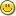### 1. Fractal Fern in Euphroia

```--====================987654321_0==_

First of all I'd like to thank everyone who sent in their sugestions about how
I should handle the attached files that ended up as giberish at the end of the
message (I've contacted HoTMail management and hope to hear what they have to
say soon.)

Here's a Fractal Fern Demo that I translated from basic to Euphoria.  Now we
all get to see what sort of problems arise when I try to attach a filefile.  In case there are problems, I've also included the file as text below.
If anyone's got any sugestions on how I can improve my Euphoria programming
techniques, I'd like to hear them.  Thanks.

----------------start code

------------Fractal Fern Demo in EUPHORIA
------------Based on a Qbasic program published in PC Magazine
------------Translated from Qbasic to Euphoria on 30 March, 1997

include graphics.e

if graphics_mode(257) then
puts(1, "You don't have mode 257\n")
elsif graphics_mode(18) then
puts(1, "Need at least VGA\n")
end if

--   These two statements from Qbasic gave me the most
--     trouble because there doesn't seem to be a Euphoria equivalent.
--VIEW (0, 0)-(639, 479)
--WINDOW (-4, 0)-(6, 10)
--     I solved this problem by changing the way the pixels --     --   were
plotted, but if anyone knows of a better way, I'd like to --   hear it.

atom A, B, C, D, E, F, R, NEWX, NEWY
sequence xy

xy = {0, 0}

puts(1, "Fractal Fern in Euphoria.\n")
puts(1, "Based on a QBasic program by PC Magazine\n")
puts(1, "Press any key to exit.\n")

while get_key() < 0 do
R = rand(100)

if R <= 10 then
A = 0
B = 0
C = 0
D = .16
E = 0
F = 0
elsif R > 1 and R <= 86 then
A = .85
B = .04
C = -.04
D = .85
E = 0
F = 1.60
elsif R > 86 and R <= 93 then
A = .2
B = -.26
C = .23
D = .22
E = 0
F = .16
else
A = -.15
B = .28
C = .26
D = .24
E = 0
F = .44
end if

NEWX = ((A * xy) + (B * xy) + E)
NEWY = ((C * xy) + (D * xy) + F)
xy = {NEWX, NEWY}

pixel(GREEN, {xy * 40 + 275, xy * 40 + 50})
-- the origional Qbasic statement was: pset(x, y), 2

end while

atom junk
junk = graphics_mode(2)
clear_screen()

----------------------------end code

---------------------------------------------------------
Get Your *Web-Based* Free Email at http://www.hotmail.com
---------------------------------------------------------

--====================987654321_0==_
Content-transfer-encoding: x-uuencode
```